• 定义指针给数组赋值
万次阅读
2018-12-18 22:55:58
#include <stdio.h>
int main(void)

{
int a={0};
int *p=a; //a代表数组首元素的首地址，即p=&a;
int i;
for(i=0;i<10;i++)
{
*(p+i)=i;
printf("a[%d]=%d\r",i,*(p+i));

}
}

结果：

a=0
a=1
a=2
a=3
a=4
a=5
a=6
a=7
a=8
a=9

更多相关内容

数组定义：

结构体定义：

typedef struct

{

int iOne;

int iTwo;

int iThr;

typedef struct

{

int iUser;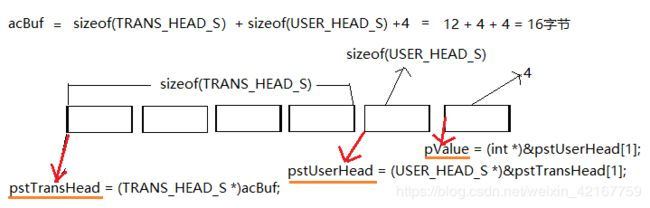1、实现结果：

acBuf = 11

acBuf = 0

acBuf = 0

acBuf = 0

acBuf = 12

acBuf = 0

acBuf = 0

acBuf = 0

acBuf = 13

acBuf = 0

acBuf = 0

acBuf = 0

acBuf = 14

acBuf = 0

acBuf = 0

acBuf = 0

acBuf = 15

acBuf = 0

acBuf = 0

acBuf = 0

2、代码实现：

#include typedef struct

{

int iOne;

int iTwo;

int iThr;

typedef struct

{

int iUser;

int main()

{

int i=0;

int *pValue = NULL;

(*pValue) = 15;

for(i = 0; i < sizeof(acBuf); i++)

{

printf("acBuf[%d] = %d\n", i, acBuf[i]);

}

return 0;

}

的解释——结构体赋值给另一个结构体

#include typedef struct

{

int iA;

int iB;

int iC;

}TEST_S;

int main(void)

{

TEST_S t1,t2;

/*给t1结构体赋值*/

t1.iA = 11;

t1.iB = 12;

t1.iC = 13;

/*t1结构体赋值给t2结构体*/

t2 = t1;

printf("t2.iA=%d t2.iB=%d t2.iC=%d\n", t2.iA, t2.iB, t2.iC);

return 0;

}

结果显示：t2.iA=11 t2.iB=12 t2.iC=13

4、char类型指针指向int类型数值,取值结果显示

#include int main()

{

int i = 1561;

char c = 0;

c = (char)i;

printf("i=%d c=%hhu\n", i, c);

return 0;

}

结果显示是：i=1561 c=25

1561：是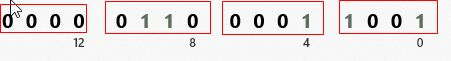25：是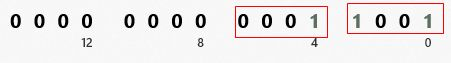展开全文• 在经过长时间的迷惑后，我放弃了使用二级指针传参 #include<stdio.h> #define N1 3 #define N2 5 void f(double (*a)[N2],double (*p)[N2]); void show(double (*p)[N2]); int main(void) { double a[N1]...

源自C primer plus P273第七题

在经过长时间的迷惑后，我放弃了使用二级指针传参

#include<stdio.h>
#define N1 3
#define N2 5
void f(double (*a)[N2],double (*p)[N2]);
void show(double (*p)[N2]);
int main(void)
{
double a[N1][N2]=
{
{1,2,3,4,5},
{6,7,8,9,10},
{11,12,13,14,15},
};
//show(a);
double b[N1][N2];
double (*p)[N2]=b;
//show(p);
//printf("%lf",(*p));
f(a,p);
show(b);
}
void f(double (*a)[N2],double (*p)[N2])
{
int i;
int j;
for(i=0;i<N1;i++)
{
for(j=0;j<N2;j++)
{
*(*(p+i)+j)=*(*(a+i)+j);
}
}
//show(a);
}

void show(double (*p)[N2])
{
int i;
int j;
for(i=0;i<N1;i++)
{
for(j=0;j<N2;j++)
{
printf("%.1lf  ",*(*(p+i)+j));
}
printf("\n");
}
}

展开全文c语言 c++ 矩阵
•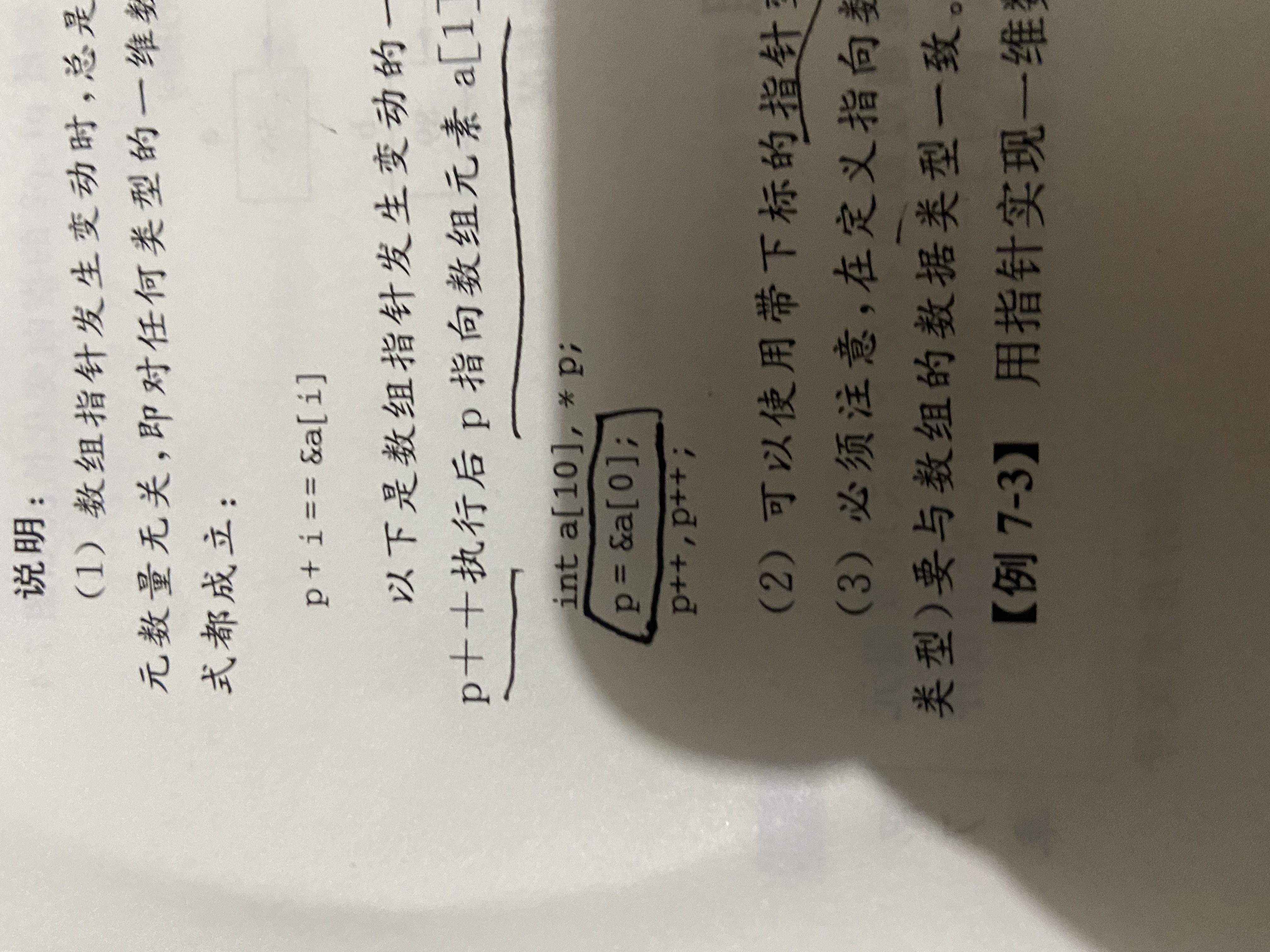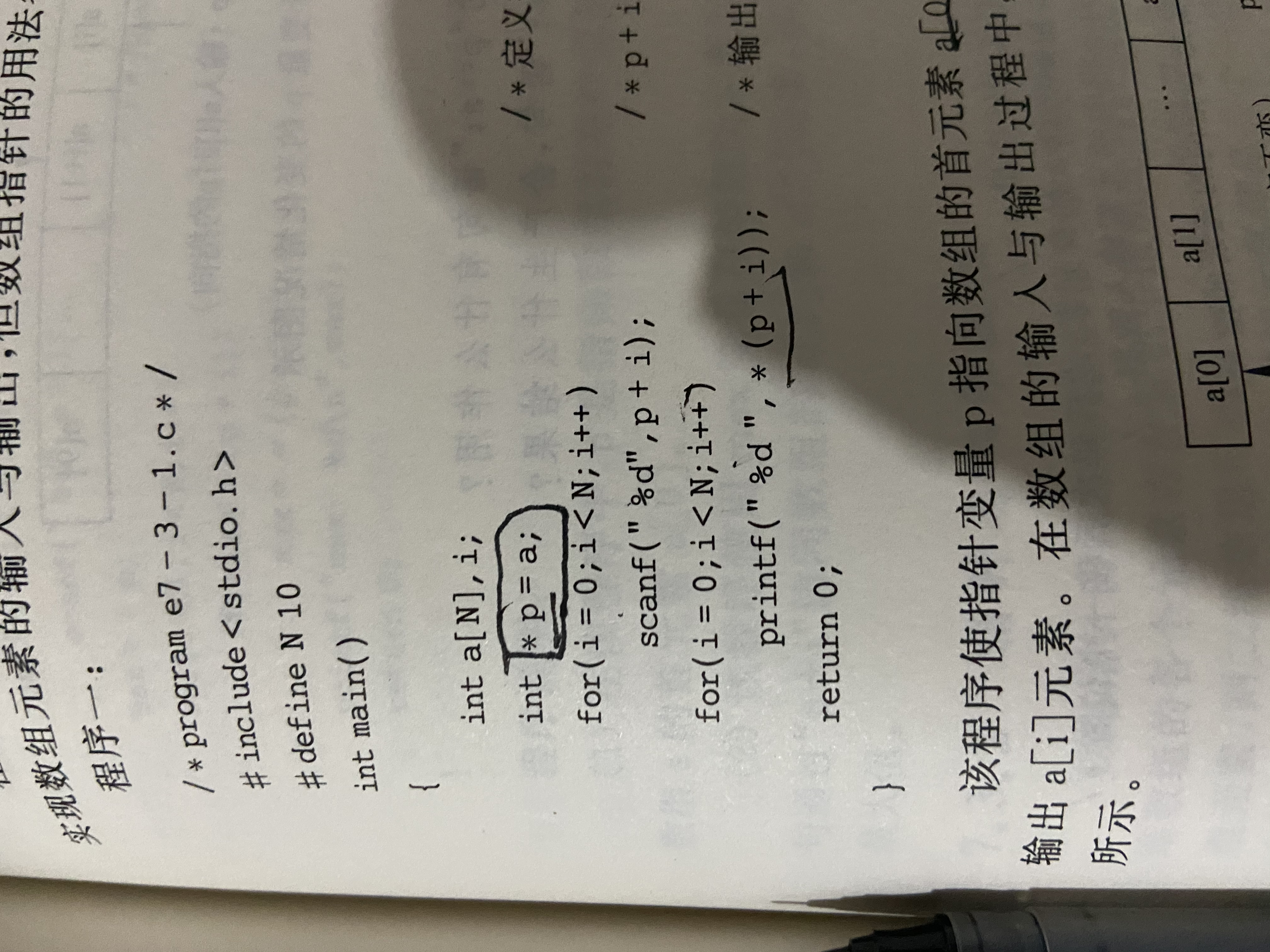展开全文• 比如 char *p=”sdflkjasljfsjlsdfsa”;...将p赋p1 （1）strcpy(p1,p); （2）char *src=”helloworld”; char des={0}; memcpy(des,src,strlen(src)+1); //void *memcpy(void *str1, const...
• c语言给数组赋值有哪些形式发布时间：2020-04-21 16:19:02来源：亿速云阅读：158作者：小新c语言给数组赋值有哪些形式？相信有很多人都不太了解，今天小编为了让大家更加了解c语言，所以大家总结了以下内容，一起...
• 问题是这样的， 我打算输入与元素为数组赋值， 然后反向输出数组元素# include int main(void) { int a, * p = a, i; for (i = 0; i ; i++) scanf("%d", p++); for(i = 9; i >= 0; i--) // 用指针输出时...
• 标题：c语言中指针数组 #include<stdio.h> #include<stdlib.h> #include<string.h> int main(){ char * arr; for(int i = 0;i < 3;i++){ arr[i] = (char *)malloc(sizeof(char *) * 10)...c语言 算法 开发语言
• 指针地址是由系统自动分配的，想要自己指定指针地址有两种方法： 一、初始化赋值 例如: char* p[] = { "Blue","Yellow","Orange","Green","Red","black" }; 此种方法很容易理解； 二、使用循环，对每个元素遍历输入...c语言 c++ 算法
• 指针和数组到底有什么样的关系，他们之间没有任何关系！指针就是指针指针变量在32 位系统下，永远占4 个byte，其值为某一个内存的地址。指针可以指向任何地方，但不是任何地方你都能通过这个指针变量访问到。数组...
• 数组给指针赋值的特殊性 变量名的地址赋值给指针时，必须加取地址符& int*p; int a=1; p=&a;//正确 p=a；//错误 数组名的地址赋值给指针时，的是首元素地址，和传递参数是一样 int arr; int *p; ...
• ## java给数组赋值

千次阅读 2021-03-23 20:12:06
java 动态数组赋值,java对象数组详解,java二维数组赋值,java给数组赋值java数组动态赋值,从零学java笔录-第24篇 图解一维数组在内存中,java二维数组赋值,java给数组赋值java 数组动态赋值,从零学java笔录-第24篇 ...
• /*给数组赋字符串*/...}上面程序在编译时, 遇到char s这条语句时, 编译程序会在内存的某处留出连续30个字节的区域, 并将第一个字节的地址赋s。当遇到strcpy( strcpy 为Turbo C2.0的函数)时, 首先在目标文件的...
• c语言给数组赋值的3种形式在C语言中，对数组进行赋值的三种形式推荐学习：C语言视频教程1、通过循环的形式 即：数组名[下标] 对数组的元素进行依次赋值#include int main(){int i;int a = {0};for(i=0;i<10;i...
• 1）数组名可以直接赋值给相同类型的指针变量 2）指针变量可以采用类似数组的用法，如：tmp.a   注：不能将数组赋值给数组变量，如： TEST jj; jj = arr; 编译将产生错误： error: ...C/C++
• 不知道行数列数的情况下，一个二维数组赋值。。。。。。c语言二维数组的赋值： 例1： inta={{80,75,92},{61,65,71},{59,63,70},{85,87,90},{76,77,85}}; 例2： #include intmain(){ inti,j;//二维数组下标 ...
• ## c中数组指针的赋值

千次阅读 2020-09-06 10:51:58
1.直接用数组名赋给指针,则指针指向数组的第一个元素的地址. 2.也可以指定数组的某一个元素,这时要加上&符号: #include <stdio.h> int main () { int var[] = {10, 100, 200}; int *ptr,*qtr; int i=1;...
• C语言中的指针数组@(C语言)[排序算法, 快速排序, C实现]引言相信指针数组是不少同学在初学C语言时遇见的大魔王。指针的的概念弄不清，导致程序写起来极易出错， 我遇见的运行错误有九成都是指针使用不当导致的，...
• 对于结构体指针，可以用来指向结构体数组的首地址，但是不能按照结构体数组赋值方式进行赋值，感觉是无法预知后面批量赋值的大小。具体情况见以下代码： #include <iostream> using namespace std; ...C++
• 1、通过循环的形式 ， 即：数组名[下标]，对数组的元素进行依次赋值 #include <stdio.h> int main() { int i; int a = {0}; for(i=0;i<10;i++) { scanf("%d",&a[i]); }c语言 开发语言
• 只有6行代码，非常简洁，这是char二级指针给char二维数组赋值的逻辑。二级指针需要先赋值操作，加断点就可以看见二维数组里的值了。
• a是一个静态数组，在C语言中其在编译期间为一个常量表达式，而常量表达式可以当做是常量使用，所以不能对于a进行指针赋值操作，既然这样是错误的，这么对于字符数组进行赋值呢 2.字符数组单个字符的赋值操作 最...c语言
• 1、数组在定义时， 可以同时进行初始化，但...可通过数组下标方式或指针方式，引用数组元素，进行赋值。 例子: char a; char ch=‘a’; for( i=0;i<10;i++ ) a[i]=ch+i ; //可通过数组下标引用数组元素，完成赋c语言
• 这是因为二维数组的初始化方式不对 错误示例： int[][] a = null; a = 0; 正确示例： int a[][] = new int; a = 0; 如果你要定义一个随机大小的数组 ...java
• 而对于动态分配的二维数组而言，b就是int**类型了，这是因为每个b[j]是一个int*类型，而这个int*又是一个一维数组，所以q=b就是错的。而它的这个特征使得在访问动态分配的二维数组的元素时，只能用b[i][j]或 * (*(b+...
• 出一段代码： #include&.../* 指针的重新赋值.c */ /* written by Chen Gengru */ /* updated on 2019-1-3 */ void main() { int i, a, b, *p, *q; p = &amp;a; q = b; pri...
• 1、指针赋值 ...//数组的首地址传指针变量，则该指针变量指向了该数组。 或者 int *p,a[i]; p=&amp;a;//数组的首地址传指针变量，则该指针变量指向了该数组。 3、二维数组指针 ......